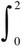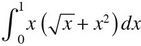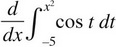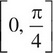# AP Calculus BC Practice Test 15

### Test Information8 questions24 minutes

Calculator Allowed

1. Use the Trapezoidal Sum to approximate(3 + x + x2)dx using n = 4 subintervals.

2. A particle moves along the x-axis so that its acceleration at any time t ≥ 0 is given by a(t) = 8sin 2t + 4cos 2t. If its initial velocity is 8 units/s and its initial position is x = 4, what is its position, in units, at time t = 2 seconds?

3.4. g(x) =5. The region in the first quadrant bounded by y = 4x - x2 and the x-axis is revolved around the x-axis. What is the volume of the resulting solid?

6. What is the length of the curve f(x) = ln(x + 1) from x = 1 to x = 3?

7. Let f(x) = 3x - x3. What is the value of c on the closed interval [1, 3] that satisfies the conclusion of the Mean Value Theorem?

8. If f(x) = arctan x, what is the average value of f(x) on the closed interval?